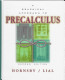# A Graphical Approach to Precalculus

Taal: Engels

## € 84,95

This book is the culmination of many years of teaching experience with the graphing calculator. In it the authors treat the standard topics of precalculus solving analytically, confirming graphically, and motivating through applications. Throughout the first five chapters, the authors present the various classes of functions studied in a standard precalculus text. Chapter One introduces functions and relations, using the linear function as the basis for the presentation. In this chapter, the authors introduce the following approach which is used throughout the next four chapters: after introducing a class of function the nature of its graph is examined, then the analytic solution of equations based on that functionis discussed. Students are then shown how to provide graphical support for solutions using a graphing calculator. Having established these two methods of solving equations, the authors move on to the analytic methods of solving the associated inequalities. Students then learn how the analytic solutions of these inequalities can also be supported graphically. Finally, once the student has a feel for the particular class of function under consideration, the authors use analytic and graphical methods to solve interesting applications involving that function. By consistently using this approach with all the different classes of functions, students become aware that the authors are always following the same general procedure, and just applying that procedure to a new kind of function. Throughout the text, the authors emphasize the power of technology but provide numerous warnings on its limitations: the authors stress that it is only through understanding the mathematical concepts that students can fully appreciate the power of graphing calculators and use technology appropriately.
ISBN
9780321028488
Vorm
Gebonden
Uitgever
Pearson Education
Druk
1e
Verschenen
02-10-1998
Taal
Engels
Pagina's
784 pp.
Genre
Literaire fictie
Geen recensies beschikbaar.